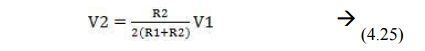Home | | High Voltage Engineering | Resistance Potential Dividers - Digital Techniques in High Voltage Measurement

# Resistance Potential Dividers - Digital Techniques in High Voltage Measurement

The resistance potential dividers are the first to appear because of their simplicity of construction, less space requirements, less weight and easy portability. These can be placed near the test object which might not always be confined to one location.

DIGITAL TECHNIQUES IN HIGH VOLTAGE MEASUREMENT

Resistance Potential Dividers

The resistance potential dividers are the first to appear because of their simplicity of construction, less space requirements, less weight and easy portability. These can be placed near the test object which might not always be confined to one location.

The length of the divider depends upon two or three factors. The maximum voltage to be measured is the first and if height is a limitation, the length can be based on a surface flash over gradient in the order of 3–4 kV/cm irrespective of whether the resistance R1 is of liquid or wire wound construction. The length also depends upon the resistance value but this is implicitly bound up with the stray capacitance of the resistance column, the product of the two (RC) giving a time constant the value of which must not exceed the duration of the wave front it is required to record. It is to be noted with caution that the resistance of the potential divider should be matched to the equivalent resistance of a given generator to obtain a given wave shape. FIG. 4.11 (a) shows a common form of resistance potential divider used for testing purposes where the wave front time of the wave is less than 1 micro sec.Figure: 4.11 various forms of resistance potential dividers recording circuits (a) Matching at  divider end (b) Matching at Oscillo graph end (c) Matching at both ends of delay cable Here R3, the resistance at the divider end of the delay cable is chosen such that R2 + R3 = Z which puts an upper limit on R2 i.e., R2 < Z. In fact, sometimes the condition for matching is given asBut, since usually R1 > > R2, the above relation reduces to Z = R3 + R2. From Fig. 4.19 (a), the voltage appearing across R2 iswhere Z1 is the equivalent impedance of R2 in parallel with (Z + R3), the surge impedance of the cable being represented by an impedance Z to ground.As this voltage wave reaches the CRO end of the delay cable, it suffers reflections as theimpedance offered by the CRO is infinite and as a result the voltage wave transmitted into the CRO is doubled. The CRO, therefore, records a voltage The reflected wave, however, as it reaches the low voltage arm of the potential divider does not suffer any reflection as Z = R2 + R3 and is totally absorbed by (R2 + R3). Since R2 is smaller than Z and Z1 is a parallel combination of R2 and (R3 + Z), Z1 is going to be smaller than R2 and since R1 > > R2, R1 will be much greater than Z1 and, therefore to a first approximation Z1 + R1 ≈ R1.Fig. 4.11 (b) and (c) are the variants of the potential divider circuit of Fig. 4.11 (a). The cable Matching is done by a pure ohmic resistance R4 = Z at the end of the delay cable and, therefore, the voltage reflection coefficient is zero i.e. the voltage at the end of the cable is transmitted completely into R4 and hence appears across the CRO plates without being reflected. As the input impedance of the delay cable is R4 = Z, this resistance is a parallel to

R2 and forms an integral part of the divider’s low voltage arm. The voltage of such a divider is, therefore, calculated as follows: Equivalent impedanceDue to the matching at the CRO end of the delay cable, the voltage does not suffer any reflection at that end and the voltage recorded by the CRO is given asFor a given applied voltage V1 this arrangement will produce a smaller deflection on the CRO Plates as compared to the one in Fig. 4.19 (a).The arrangement of Fig. 4.19 (c) provides for matching at both ends of the delay cable and is to be recommended where it is felt necessary to reduce to the minimum irregularities produced in the delay cable circuit. Since matching is provided at the CRO end of the delay cable, therefore, there is no reflection of the voltage at that end and the voltage recorded will be half of that recorded in the arrangement of Fig. 4.19 (a) vizIt is desirable to enclose the low voltage resistance (s) of the potential dividers in a metal screening box. Steel sheet is a suitable material for this box which could be provided with a detachable closefitting lid for easy access. If there are two low voltage resistors at the divider position as in Fig. 4.11 (a) and (c), they should be contained in the screening box, as close together as possible, with a removable metallic partition between them. The partition serves two

purposes (i) it acts as an electrostatic shield between the two resistors (ii) it facilitates the changing of the resistors. The lengths of the leads should be short so that practically no inductance  is  contributed  by these  leads.  The  screening  box  should  be  fitted  with  a  large earthling terminal. Fig. 4.12 shows a sketched cross-section of possible layout for the low voltage arm of voltage divider.Study Material, Lecturing Notes, Assignment, Reference, Wiki description explanation, brief detail
High Voltage Engineering : Measurement of High Voltage and High Currents : Resistance Potential Dividers - Digital Techniques in High Voltage Measurement |